Maths-
General
Easy

Question

# A conical vessel of height 10ft and semivertical angle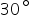is full of water. If empties in such away that the height at the water in the vessel is decreasing at a constant rate of 1ft/min. Then the rate at which the volume of water in the vessel is decreasing when its height is  6ft. is

##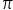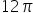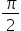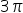Hint:

## The correct answer is:###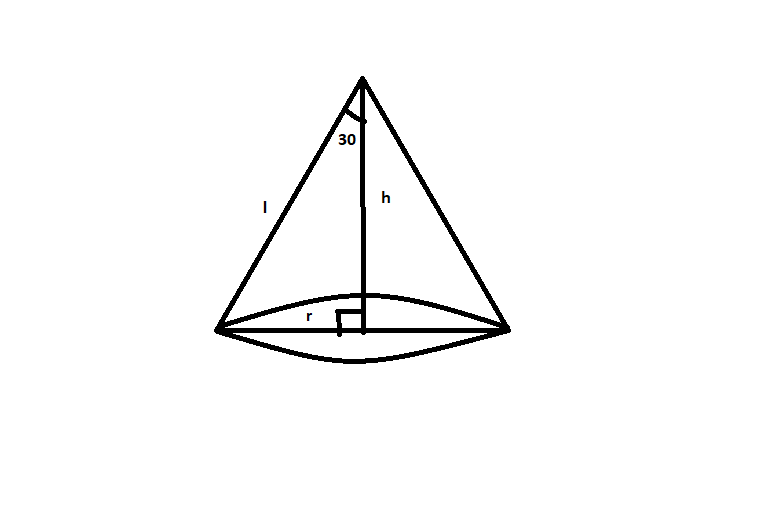Given :We know that, tan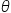=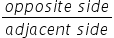tan=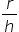Volume of conical vessel (V) =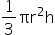V =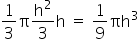Differentiating the above wrt to timeThus, the rate at which the volume of water in the vessel is decreasing when its height is  6ft. is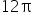#### With Turito Foundation.#### Get an Expert Advice From Turito.